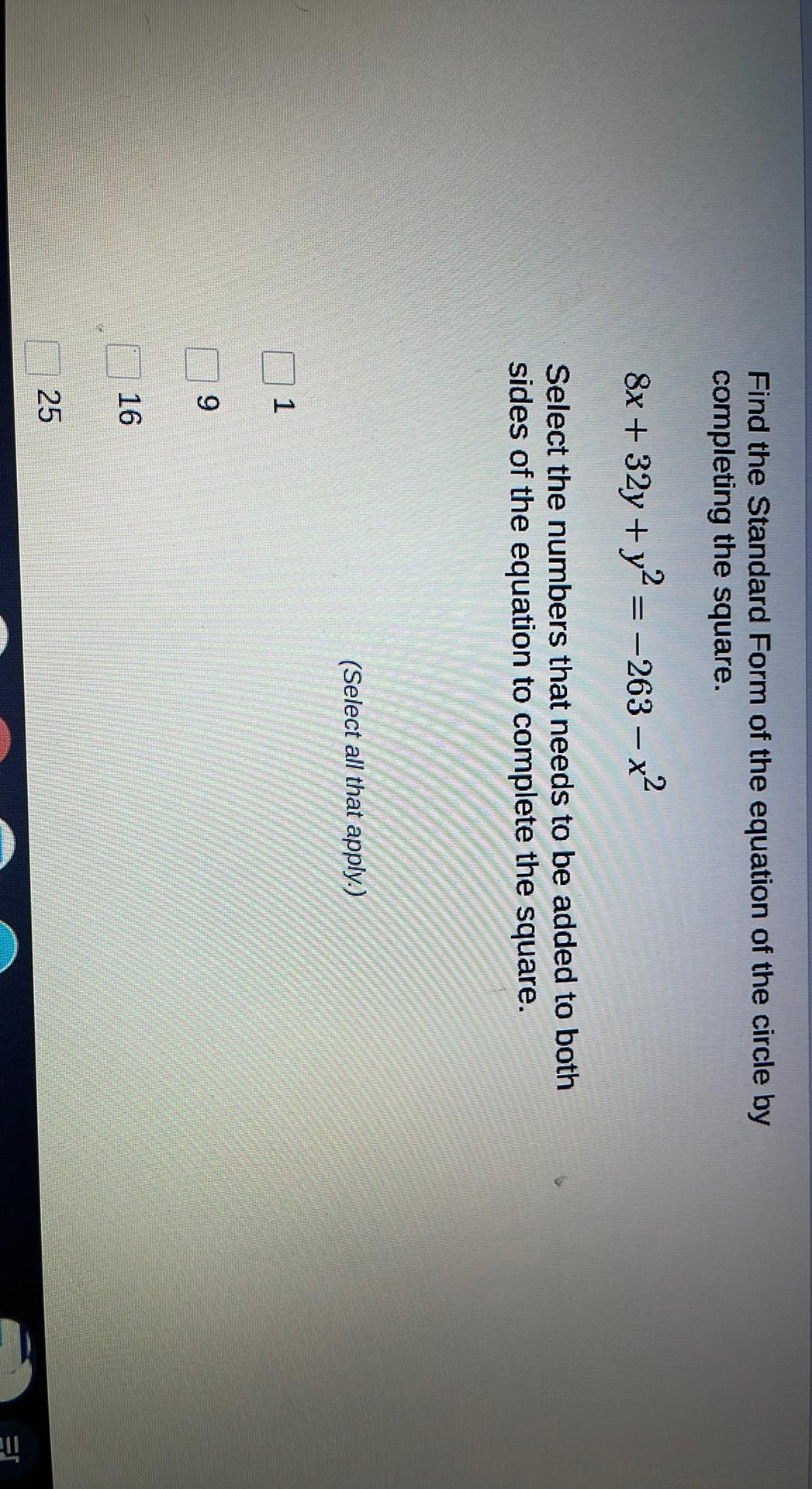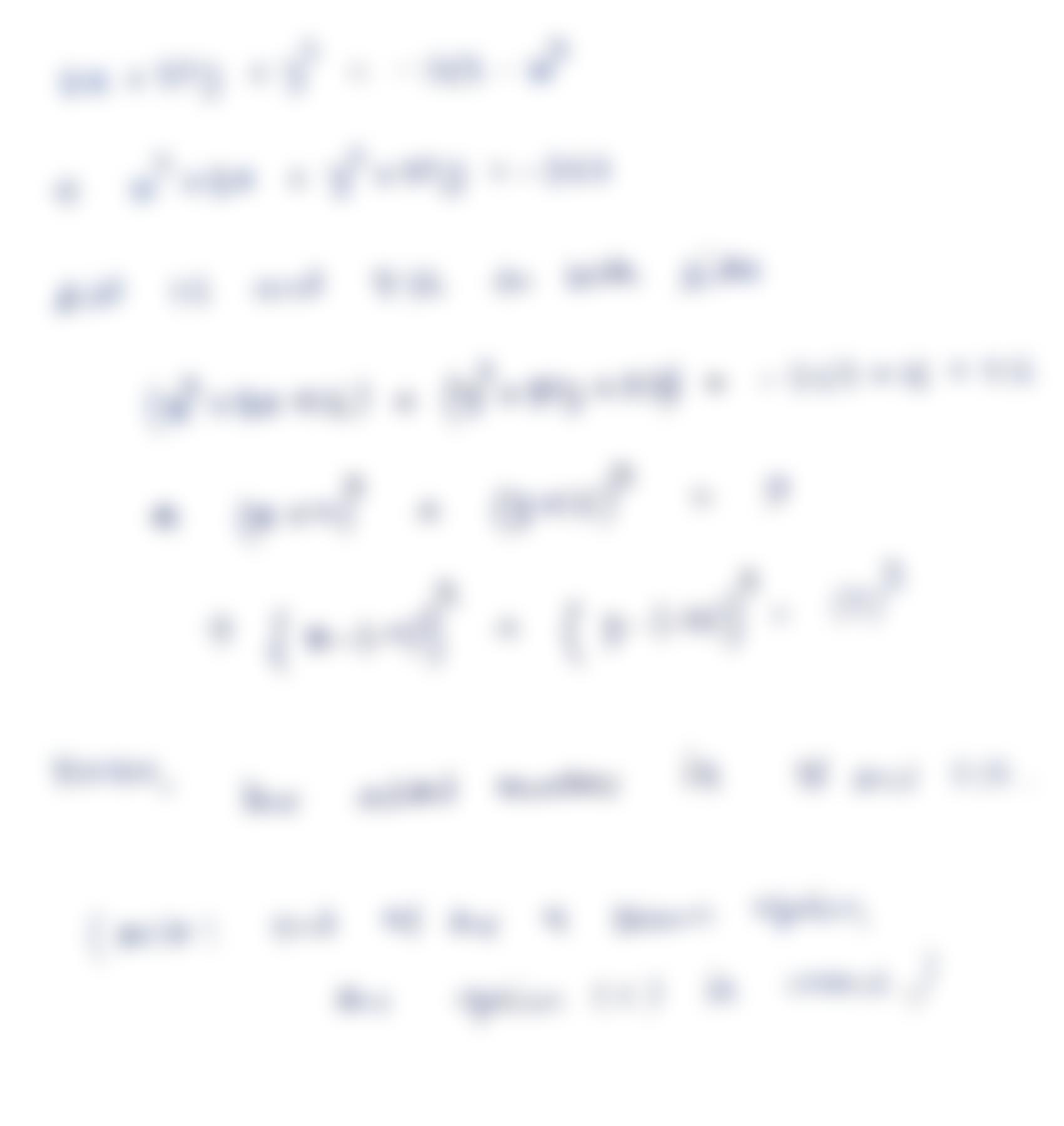Question:

Find the Standard Form of the equation of the circle by completing the square. 8x + 32y + y2 = -263 – x2 - - X Select the numberFind the Standard Form of the equation of the circle by completing the square. 8x + 32y + y2 = -263 – x2 - - X Select the numbers that needs to be added to both sides of the equation to complete the square. (Select all that apply.) 1 9 16 25